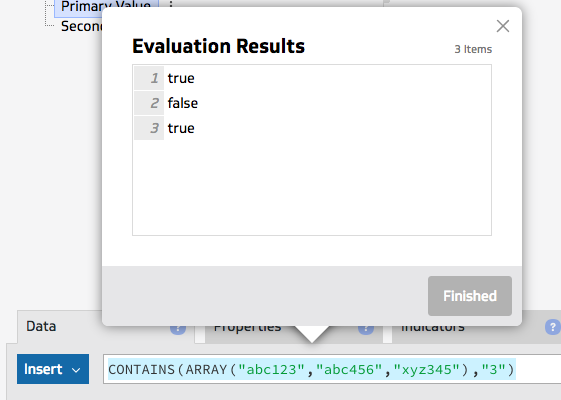I have a field that contains text and numbers.  I want to return a Boolean where if the row contains a number, then it should be TRUE, else FALSE.  Any suggestions?

•Rahul Bura

Hey Vincent,

Thanks for your post! You can use the CONTAINS function in this case - you can see the example below. I'm checking to see if items in an array, the entries contain a 3 and you can see the list of TRUE's and FALSE's.•Larona Nyambe

Hello Vincent,

Adding to Rahul's answer. If what you meant is you have a field which returns numbers or text and you want to return a boolean for each case. E.g If your field returns [10,20,abc,e,2] you want to return [true,true,false,false,true]. Then one solution can be :

`SLICE(OR(NUMBERFORMAT(ABS(ARRAY(BLANK(),<data>)))>0,ARRAY(BLANK(),<data>)=0))`

The formula is a bit lengthy because I am trying to cover for all the conditions, if you know your data set you can simplify the formula. E.g if there will never be a negative number remove the ABS() function.

If these answers don't work for you, feel free to contact us at support@klipfolio.com and we will be happy to take a look at your klip.

Cheers,

L.N